# Texas Go Math Grade 3 Lesson 8.3 Answer Key Patterns on the Multiplication Table

Refer to our Texas Go Math Grade 3 Answer Key Pdf to score good marks in the exams. Test yourself by practicing the problems from Texas Go Math Grade 3 Lesson 8.3 Answer Key Patterns on the Multiplication Table.

## Texas Go Math Grade 3 Lesson 8.3 Answer Key Patterns on the Multiplication Table

Essential Question
How can you use properties to explain patterns on the multiplication table?

Unlock the Problem
You can use a multiplication table to explore number patterns.

Activity 1
Materials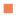Math Board
• Write the products for the green squares. What do you notice about the products?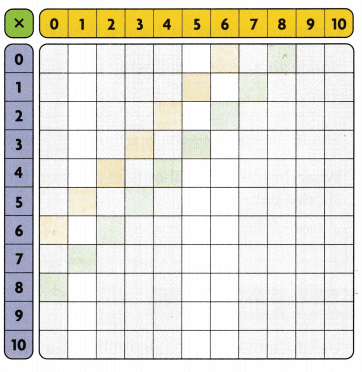Write the multiplication sentences for the products on your MathBoard. ‘ What do you notice about the factors?

• Will this be true in the yellow squares? Explain using a property you know.

Write the products for the yellow squares.

• Complete the columns for 1, 5, and 6. Look across each row and compare the products. What do you notice?

What property does this show?

Math Talk
Mathematical Processes
Explain how you can use these patterns to find other products.

Activity 2
Materials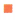yellow and blue crayons

• Shade the rows for 0, 2, 4, 6, 8, and 10 yellow.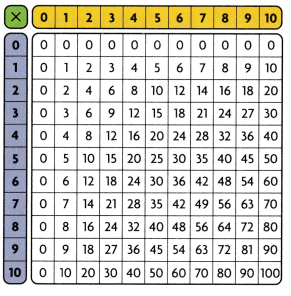• Shade the columns for 1, 3, 5, 7, and 9 blue.
• What do you notice about the products for each shaded column?

Remember
Even numbers end in 0, 2, 4, 6, or 8. Odd numbers end in 1, 3, 5, 7, or 9.

Share and Show

Question 1.
Use the table to write the products for the row for 2.
__, __, __, __, ___, ___
__, __, __, __, ___, ___, ___
Describe a pattern you see.
Product for row 2: 0, 2, 4, 6, 8, 10, 12, 14, 16, 18, 20. It is noticed that  all numbers of products of 2 are all even numbers.

Explanation:
Product for row 2:
2 × 0 = 0.
2 × 1 = 2.
2 × 2 = 4.
2 × 3 = 6.
2 × 4 = 8.
2 × 5 = 10.
2 × 6 = 12.
2 × 7 = 14.
2 × 8 = 16.
2 × 9 = 18.
2 × 10 = 20.

Math Talk
Mathematical Processes
What do you notice about the product of any number and 2?
It is noticed that any number odd or even multiplied with 2 number, their product is even number not odd number.

Explanation:
It is noticed that any number odd or even multiplied with 2 number, their product is even number not odd number.

Is the product even or odd? Write even or odd.

Question 2.
5 × 8 ___
5 × 8 = 40.(Even number)

Explanation:
Product:
5 × 8  = 40.(ends with 0 => Even number)

6 × 3 __
6 × 3 = 18.(Even number)

Explanation:
Product:
6 × 3 = 18(ends with 8 => Even number)

Question 4..

3 × 5 ___
3 × 5 = 15. (Odd number)

Explanation:
Product:
3 × 5 = 15. (ends with 5 => Odd number)

Question 5.
4 × 4 ____
4 × 4 = 16.(Even number)

Explanation:
Product:
4 × 4 = 16.(ends with 6 => Even number)

Use the multiplication table. Describe a pattern you see.
Question 6.
in the column for 10
It is noticed that pattern in column for 10 is 0, 10, 20, 30, 40, 50, 60, 70, 80, 90, 100.

Explanation:
Pattern in column for 10:
0 × 10 = 0.
1 × 10 = 10.
2 × 10 = 20.
3 × 10 = 30.
4 × 10 = 40.
5 × 10 = 50.
6 × 10 = 60.
7 × 10 = 70.
8 × 10 = 80.
9× 10 = 90.
10 × 10 = 100.

in the column for 8
It is noticed that the pattern in a column for 8 is 0, 8, 16, 24, 32, 48, 56, 64, 72, 80.

Explanation:
the pattern in a column 8:
0 × 1 = 0.
8 × 1 = 8.
8 × 2 = 16.
8 × 3 = 24.
8 × 4 = 32.
8 × 5 = 40.
8 × 6 = 48.
8 × 7 = 56.
8 × 8 = 64.
8× 9 = 72.
8 × 10 = 80.

Problem Solving
Complete the table. Then describe a pattern you see in the products.
Question 8.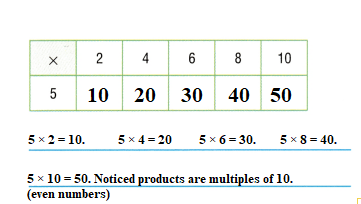Explanation:
Products:
5 × 2 = 10.
5 × 4 = 20.
5 × 6 = 30.
5 × 8 = 40.
5 × 10 = 50.
Noticed products are multiples of 10 and are even numbers.

Question 9.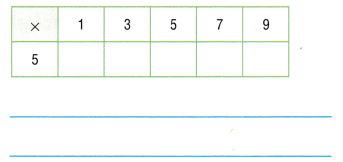Explanation:
Products:
5 × 1= 5.
5 × 3 = 15.
5 × 5 = 25.
5 × 7 = 35.
5 × 9 = 45.
Noticed products are multiples of 5 and are odd numbers.

Go Math Lesson 8.3 3rd Grade Patterns in the Multiplication Table Question 10.
H.O.T. Write Math Explain how patterns of the ones digits in the products relate to the factors in Exercises 8 and 9.
It is noticed that patterns of the ones digits in the products relate to the factors in Exercises 8 and 9, they are odd numbers when 5 number is multiplied with , 3, 5, 7 ,9 products are 5, 15, 25, 35, 45 and even numbers when multiplied by 0, 2, 4, 6, 8 and 10 products are 0, 10, 20, 30, 40, 50.

Explanation:
Noticed that patterns of the ones digits in the products relate to the factors in Exercises 8 and 9, they are odd numbers when 5 number is multiplied with , 3, 5, 7 ,9 products are 5, 15, 25, 35, 45 and even numbers when multiplied by 0, 2, 4, 6, 8 and 10 products are 0, 10, 20, 30, 40, 50.

H.O.T. Multi-Step Sense or Nonsense?

Question 11.
Elijah says the product of an odd number and an even number is even. Chloe says the product of two even numbers is even. Whose statement makes sense? Whose statement is nonsense? Use Math Language to explain your reasoning.Both statements are true and correct because the product of an odd number and an even number is even and the product of two even numbers is even.

Explanation:
Elijah says the product of an odd number and an even number is even. Chloe says the product of two even numbers is even. Both statements are true and correct.

Fill in the bubble for the correct answer choice.
Question 12.
A spider has 8 legs. Josie is using the multiplication table to find the number of legs on 1, 2, 3, 4, 5, and 6 spiders.
Which pattern do the products show?(A) odd, then even, then repeat
(B) even, then odd, then repeat
(C) all odd
(D) all even
Products:  5, 16, 24, 32, 40, 48 are all even numbers.(ending with 0,2,4,6,8)
(D) all even.

Explanation:
Product:
8 × 1 = 8.
8 × 2 = 16.
8 × 3 = 24.
8 × 4 = 32.
8 × 5 = 40.
8 × 6 = 48.

Question 13.
Xavier is counting groups of students at tables in the lunchroom. Which describes Xavier’s pattern?
6, 12, 18, 24, 30, 36
(A) Multiply by 2.
(B) Multiply by 3.
6, 12, 18, 24, 30, 36.
It is noticed they are multiples of 6 or add of 6.

Explanation:
6 × 1 = 6.
6 × 2 = 12.(6 + 6)
6 × 3 = 18.(12 + 6)
6 × 4 = 24.(18 + 6)
6 × 5 = 30.(24 + 6)
6 × 6 = 36.(30 + 6)

Multi-Step What are the next 2 numbers in this pattern?
7, 14, 21, 28, 35, ?, ?
(A) 37, 39
(B) 42, 49
(C) 70, 80
(D) 49, 56
7, 14, 21, 28, 35, ?, ?
Missing numbers are 42 and 49.
(B) 42, 49.

Explanation:
7, 14, 21, 28, 35, ?, ?
=> 7 + 7 = 14.
14 + 7 = 21.
21 + 7 = 28.
28 + 7 = 35.
35 + 7 = 42.
42 + 7 = 49.

Texas Test Prep
Question 15.
Which of the following describes this pattern?
12, 16, 20, 24, 28
(A) Multiply by 4.
(C) Multiply by 5.
(D) subtract 4.
12, 16, 20, 24, 28 are multiples of 4.
(A) Multiply by 4.

Explanation:
12, 16, 20, 24, 28.
4 × 1 = 4.(0 + 4 = 4)
4 × 2 = 8.(4 + 4 = 8)
4 × 3 = 12.(8 + 4 = 12)
4 × 4 = 16.(12 + 4= 16)
4 × 5 = 20.(16 + 4 = 20)
4 × 6 = 24.(20 + 4 = 24)
4 × 7 = 28.(24 + 4 = 28)

### Texas Go Math Grade 3 Lesson 8.3 Homework and Practice Answer Key

Use the multiplication table. Describe a pattern you see.
Question 1.
in the column for 4Product in the column 4: 0, 4, 8, 12, 16, 20, 24, 28, 32, 36, 40
It is noticed they are multiples of 4 and all are even numbers.

Explanation:
Product in the column 4:
4 × 0 = 0.
4 × 1 = 4.
4 × 2 = 8.
4 × 3 = 12.
4 × 4 = 16.
4 × 5 = 20.
4× 6 = 24.
4 × 7 = 28.
4 × 8 = 32.
4 × 9 = 36.
4 × 10 = 40.

Go Math 3rd Grade Practice and Homework Lesson 8.3 Answer Key Question 2.
in the column for 5
Product in the column 5: 0, 5, 10, 15, 20, 25, 30, 35, 40, 45, 50.
It is noticed they are multiples of 5 and numbers 5, 15, 25, 35, 45 are odd numbers and 0, 10, 20, 30, 40, 50 are even numbers(multiples of 10).

Explanation:
Product in the column 5:
5 × 0 = 0.
5 × 1 = 5.
5 × 2 = 10.
5 × 3 = 15.
5 × 4 = 20.
5 × 5 = 25.
5× 6 = 30.
5 × 7 = 35.
5 × 8 = 40.
5 × 9 = 45.
5 × 10 = 50.

Complete the table. Then describe a pattern you see in the products.
Question 3.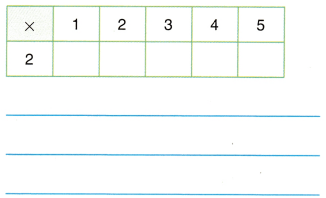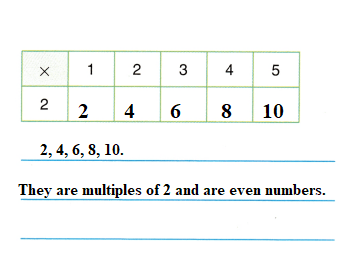Explanation:
2 × 1 = 2.
2 × 2 = 4.
2 × 3 = 6.
2 × 4 = 8.
2 × 5 = 10.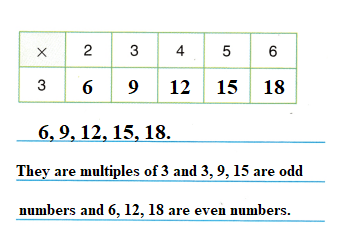Explanation:
3 × 1 = 3.
3 × 2 = 6.
3 × 3 = 9.
3 × 4 = 12.
3 × 5 = 15.
3 × 6 = 18.

Problem Solving
Question 5.
Lani says that the product of an odd number and an odd number is an odd number. Draw a picture and write a number sentence that shows how Lani’s statement makes sense.
Lani’s statement makes sense because the product of an odd number and an odd number is an odd number.
Total number of stars = 3 × 5 = 15.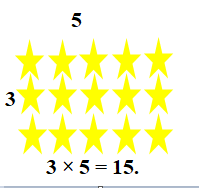Explanation:
The product of an odd number and an odd number is an odd number:
3 × 5 = 15.Number of rows of stars = 3.
Number of columns of stars = 5.
Total number of stars = Number of rows of stars × Number of columns of stars
= 3 × 5
= 15.

Texas Test Prep
Lesson Check
Question 6.
A store sells tricycles. Each tricycle has 3 wheels. Jin uses a multiplication table to find the total number of wheels. Which pattern do the products show?(A) all odd
(B) all even
(C) odd, then even, then repeat
(D) even, then odd, then repeat
6, 12, 18, 24, 30 are all even numbers.
(B) all even.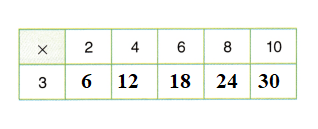Explanation:
3 × 1 = 3.
3 × 2 = 6.
3 × 4 = 12.
3 × 6 = 18.
3 × 8 = 24.
3 × 10 = 30.

Jin also uses a multiplication table to find the total number of wheels on the bicycles in the store. Which pattern do the products show?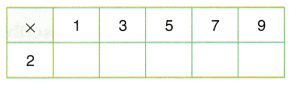(A) all odd
(B) all even
(C) odd, then even, then repeat
(D) odd, even, odd, then repeat
2,  6, 10, 14, 18 are even numbers and multiples of 2.
(B) all even.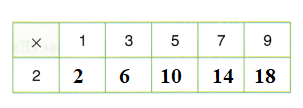Explanation:
2 × 1 = 2.
2 × 3 = 6.
2 × 5 = 10.
2 × 7 = 14.
2 × 9 = 18.

Question 8.
Matt is counting groups of squares on a quilt he is making. Which describes Matt’s pattern?
3, 6, 9, 12, 15, 18
(A) Skip count by 2.
(B) Multiply by 3.
3, 6, 9, 12, 15, 18.
They are the multiples of 3 and add of 3.
(B) Multiply by 3.

Explanation:
3, 6, 9, 12, 15, 18
3 × 1 = 3.(0 + 3 = 3)
3 × 2 = 6.(3 + 3 = 6)
3 × 3 = 9.(6 + 3 = 9)
3 × 4 = 12.(9 + 3 = 12)
3 × 5 = 15.(12 + 3 = 15)
3 × 6 = 18.(15 + 3 = 18)

Question 9.
Cassie is counting groups of beads on a necklace. Which describes Cassie’s pattern?
4, 9, 14, 19, 24, 29
(B) Multiply by 4.
(C) Multiply by 5.
4, 9, 14, 19, 24, 29.
They are the add of 5.

Explanation:
4, 9, 14, 19, 24, 29.
4 + 5 = 9.
9 + 5 = 14.
14 + 5 = 19.
19 + 5 = 24.
24 + 5 = 29.

Multi-Step lacy makes a pattern with triangles. How many triangles does Jacy need to place in the next two parts of his pattern?
4, 10, 16, 22, 28, ?, ?
(A) 29, 30
(B) 34, 40
(C) 32, 36
(D) 30, 32
4, 10, 16, 22, 28, ?, ?
34, 40 triangles Jacy needs to place in the next two parts of his pattern.
(B) 34, 40.

Explanation:
4, 10, 16, 22, 28, ?, ?
4 + 6 = 10
10 + 6 = 16.
16 + 6 = 22.
22 + 6 = 28.
28 + 6 = 34.
34 + 6 = 40.

Question 11.
Multi-Step Vito makes a pattern with puzzle pieces. How many puzzle pieces does Vito have in the first part of the pattern?
?, ?, 8, 11, 14, 17, 20
(A) 2, 4
(B) 1, 3
(C) 2, 5
(D) 3, 5
?, ?, 8, 11, 14, 17, 20
2, 5 are puzzle pieces Vito have in the first part of the pattern.
(C) 2, 5.

Explanation:
?, ?, 8, 11, 14, 17, 20.
20 – 17 = 3.
17 – 3 = 14.
14 – 3 = 11.
11 – 3 = 8.
8 – 3 = 5.
5 – 3 = 2.

Scroll to Top